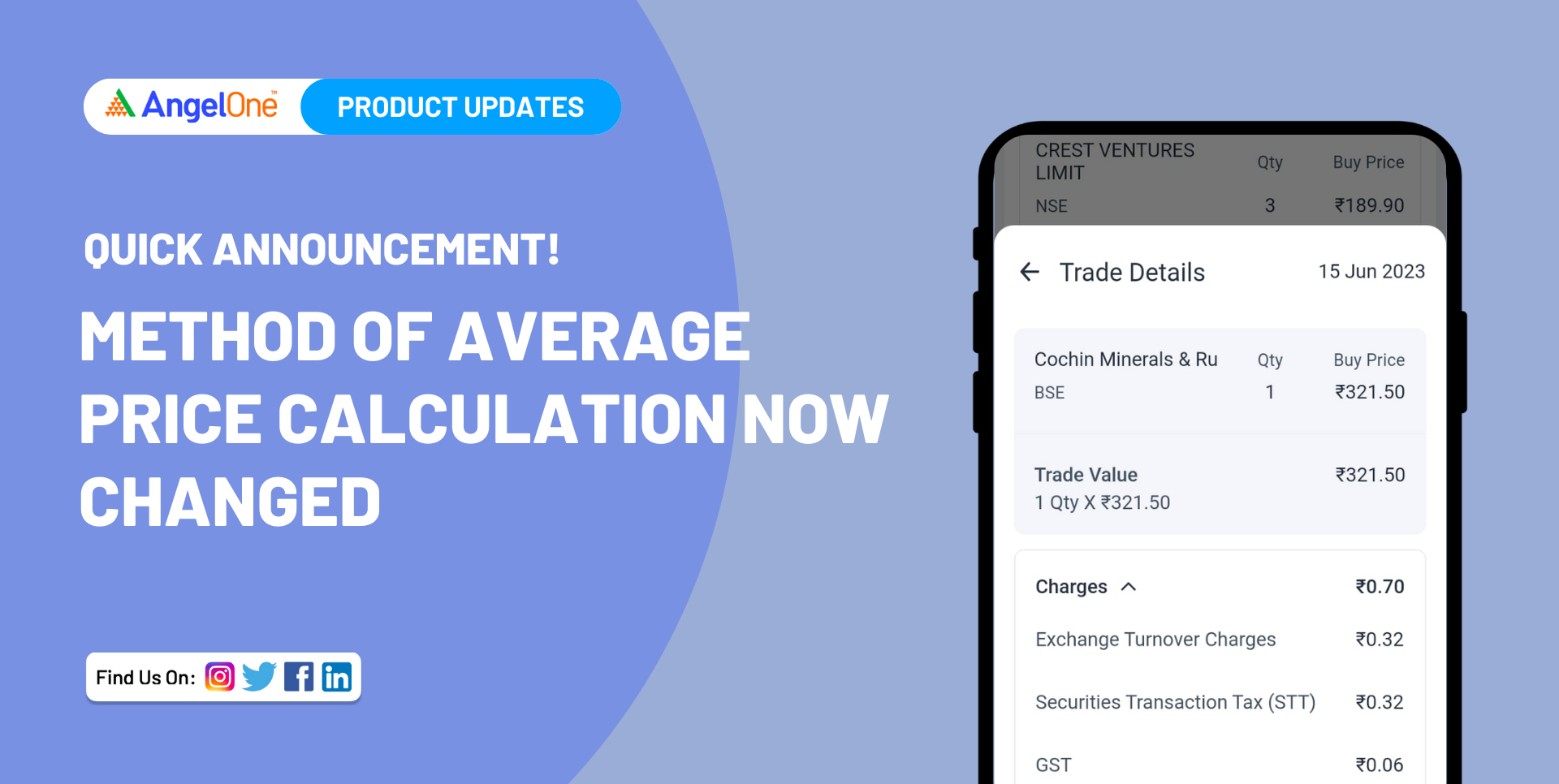# Method of Average Price Calculation Now Changed

16 June 2023
by Angel One
Average prices of commodity derivatives displayed on the Angel One app are now more accurate and transparent.One of the biggest assets of any fintech company is the trust that it enjoys among its users. This trust is in turn, based on the transparency, performance and responsibility displayed by the company.

With these principles in mind, we, at Angel One, decided to change the way the average price for commodity derivatives is calculated.

## What is Average Price?

A trader or an investor may buy or sell the same asset multiple times over a period. These trades will probably occur at different prices. In such a situation, we can find the average price at which the asset was bought to fully understand the level of profit earned by the trader.

This is accomplished by using the following formula,

A =  I∕S

Where,

A is the Average Price

I is the total investment

S is the total number of assets bought

## What is New?

To better understand the changes in average price calculation, let us look at the process of how the average price is now calculated for all derivatives on the Angel One app:

1. On the first day (or T day), the average price seen in the position book is exclusive of all charges.
2. At the end of the trading day (or T day), the billing of the day’s trades is done based on a table of transactions. This table becomes a reliable source for the average price calculation.
3. On the next day (i.e. T+1 day), we recalculate the average price of the open position. This figure is, however, inclusive of all the charges

Now, in the new system, the brokerage (alongside other charges) will also be included while calculating the average price for commodity derivatives.

This will slightly increase the prices of commodity derivatives.

Let us understand this process with an example.

Example of Average Price Calculation

Suppose you have invested Rs. 1,00,000 to buy 100 shares. Assume the brokerage stands at Rs. 20 and other charges at Rs. 300.

Therefore,

Average price on T day = Total invested value∕Total quantity  =  1,00,000∕100  =  Rs. 1000

Under the old system,

Average price on T+1 day  =  (1,00,000 + 300)∕100 =  Rs. 1003

The total invested value would have been considered as Rs. 1,00,300.

Under the new system,

Average price on T+1 day =  (1,00,000 + 20 + 300)∕100  =  1003.2

The total invested value would be considered to be Rs. 1,00,320.

This is because the brokerage of Rs. 20 is now also considered a part of the total invested value.

For reference, below is a full list of the charges applicable.

## List of Charges Included

The different charges that become a part of the average price are:

1. Brokerage
2. STT
3. Turnover tax
4. GST
5. Stamp Duty
6. Exchange service tax
7. Other charges

## Benefits of the New Process

With the new source, we have improved the following:

1. Greater reliability – As we are picking charges from the very source of the contract note the price shown is a reliable figure.
2. Corporate Action Handling –  The zero average price issue in the case of corporate action is solved. The user will now see the actual invested price.
3. Brokerage will now be included when calculating the average price of commodity position. It will tell users exactly how much cash is being spent behind every trade.

The above changes for F&O and Currency segments already. It is soon going to be introduced for the commodity segment as well!

## Conclusion

The new process in average price calculation is more logical as well as reliable. If you are interested in more such product features and stock market trading, join the Angel One community today!

## FAQ

### How is average price calculated on Angel One?

On the first day (or T day), the average price seen in the position book is exclusive of all charges. At the end of the day, the day’s transactions and charges are calculated. On the next day (i.e. T+1 day), we recalculate the average price of the open position. This figure is inclusive of all the charges

### What is the average price formula?

The average price formula is the following:

Average price = Total invested value∕Total quantity